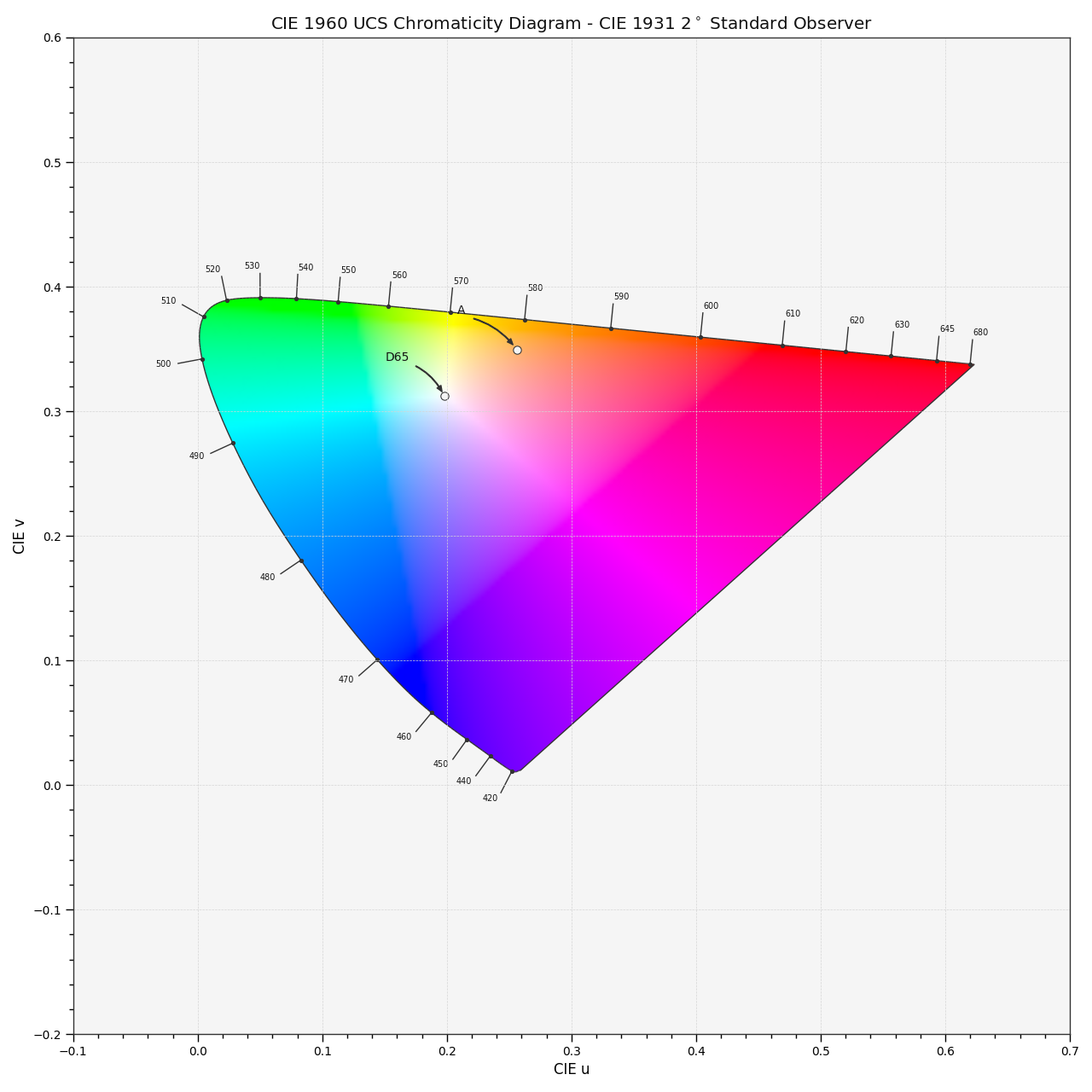# colour.plotting.plot_sds_in_chromaticity_diagram_CIE1960UCS¶

colour.plotting.plot_sds_in_chromaticity_diagram_CIE1960UCS(sds: , cmfs: = 'CIE 1931 2 Degree Standard Observer', chromaticity_diagram_callable_CIE1960UCS: Callable = plot_chromaticity_diagram_CIE1960UCS, annotate_kwargs: Optional[Union[Dict, List[Dict]]] = None, plot_kwargs: Optional[Union[Dict, List[Dict]]] = None, **kwargs: Any) [source]

Plot given spectral distribution chromaticity coordinates into the CIE 1960 UCS Chromaticity Diagram.

Parameters
Returns

Current figure and axes.

Return type

`tuple`

Examples

```>>> A = SDS_ILLUMINANTS['A']
>>> D65 = SDS_ILLUMINANTS['D65']
>>> plot_sds_in_chromaticity_diagram_CIE1960UCS([A, D65])
...
(<Figure size ... with 1 Axes>, <...AxesSubplot...>)
```